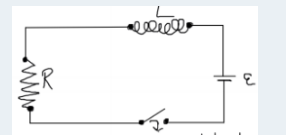Physics Practice Problems LR Circuits Practice Problems Solution: An inductor with an inductance of L = 6.0 H and a ...

# Solution: An inductor with an inductance of L = 6.0 H and a resistor with resistance R = 10 Ω are connected in series to the terminals of a battery with EMF = 20 V and negligible internal resistance. There is a switch inserted in the circuit in line between battery and the inductor: Find the current 0.015 seconds after the switch is closed.

###### Problem

An inductor with an inductance of L = 6.0 H and a resistor with resistance R = 10 Ω are connected in series to the terminals of a battery with EMF = 20 V and negligible internal resistance. There is a switch inserted in the circuit in line between battery and the inductor: Find the current 0.015 seconds after the switch is closed.LR Circuits

LR Circuits

#### Q. In the following circuit, the battery has a voltage of 40 V. At some instant in time, the voltage across the resistor is found to be 10 V. At this ins...

Solved • Wed May 10 2017 11:07:11 GMT-0400 (EDT)

LR Circuits

#### Q. Consider the circuit shown in the sketch. Initially the switch is open and no currents flow.  After the switch has been closed a long time, it is ope...

Solved • Thu Jan 26 2017 09:46:16 GMT-0500 (EST)

LR Circuits

#### Q. Consider the circuit shown in the sketch. The switch has been closed for a long time and then it is opened. Just after the switch is opened, the curre...

Solved • Tue Jan 24 2017 10:30:16 GMT-0500 (EST)

LR Circuits

#### Q. Consider the circuit shown in the sketch. The battery emf is 48.0 V,  R1 = 16.0 Ω, and R2 = 6.00 Ω. Initially no currents are flowing. Then the switch...

Solved • Mon Jan 23 2017 11:02:58 GMT-0500 (EST)